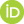Dataset Open Access

# LDOS/SNAP data for MALA: Beryllium at 298KFiedler, Lenz;Cangi, Attila

Beryllium data set for Machine Learning applications


This dataset contains DFT inputs, outputs, LDOS data and fingerprint vectors for a beryllium cell at ambient conditions and varying sizes. Different levels of k-grid convergence were employed:
-  Gamma point (gamma_point)
-  total energy convergence (k-grid converged to 1meV/atom to total energy difference, total_energy_convergence)
-  LDOS convergence (k-grid converged to LDOS without unphyiscal oscillations, ldos_convergence)

The data set contains a .zip file for each system size (see below), as well as one .zip file containing sample scripts for recalculation and preprocessing of data.
The cutoff energy was converged with respect to the energy convergence and held fixed 40Ry for all three levels of k-grids. Note that not for all sizes of unit cells data for all types of k-grid were generated.

Authors:

- Fiedler, Lenz (HZDR / CASUS)
- Cangi, Attila (HZDR / CASUS)

Affiliations:

HZDR - Helmholtz-Zentrum Dresden-Rossendorf

CASUS - Center for Advanced Systems Understanding

Dataset description

- Total size: 143G GB
- System: Be128, Be256, Be512, Be1024, Be2048
- Temperature(s): 298K
- Mass density(ies): 1.896 gcc
- Crystal Structure: hpc (material mp-87 in the materials project)
- Number of atomic snapshots: 145
- 40 (Be128)
- 35 (Be256)
- 30 (Be512)
- 20 (Be1024)
- 10 (Be2048)
- Contents:
- ideal crystal structure: yes
- MD trajectory: yes
- Atomic positions: yes
- DFT inputs: yes
- DFT outputs (energies): yes
- SNAP vectors: yes (partially, see below)
- dimensions: XxYxZx94 (last dimension: first three entries are x,y,z coordinates, data size is 91), where X, Y, Z are:
- Be128: 72x72x120 (size per file: 447MB)
- Be256: 144x72x120  (size per file: 893MB)
- Be512: 144x144x120 (size per file: 1.8GB)
- units: a.u./Bohr
- LDOS vectors: yes (partially, see below)
- dimensions: XxYxZx250, where X, Y, Z are:
- Be128: 72x72x120 (size per file: 1.2GB)
- Be256: 144x72x120  (size per file: 2.4GB)
- Be512: 144x144x120 (size per file: 4.7GB)
- units: 1/eV
- note: LDOS parameters are the same for all sizes of the unit cell
- trained networks: no

Data generation

Ideal crystal structures were obtained using the Materials Project. (https://materialsproject.org/materials/mp-87/)
DFT-MD calculations were performed using either QuantumESPRESSO (https://www.quantum-espresso.org/, QE, for Be128, Be256 and Be512) or the Vienna Ab initio Simulation Package (https://www.vasp.at/, VASP, for Be1024, Be2048). DFT calculations were performed using QuantumESPRESSO.
For the VASP calculations, the standard VASP pseudopotentials were used. For Quantum Espresso, pslibrary was used (https://dalcorso.github.io/pslibrary/).
SNAP vectors were calculated using MALA (https://github.com/mala-project/mala) and its LAMMPS (https://github.com/mala-project/mala) interface. The LDOS was preprocessed using MALA as well.

Dataset structure

The folder called "sample_inputs" is provided to show how MALA preprocessing and LDOS calculation have been performed.
For each temperature/mass density/number of atoms, the following subfolders exist:

- md_inputs: Input files for the MD simulations, either as QE or VASP file(s)
- md_outputs: The MD trajectory plus a numpy array containing the temperatures at the individual time steps
- gamma_point
- total_energy_convergence
- ldos_convergence

Each gamma_point/total_energy_convergence/ldos_convergence contains the following folders:

- ldos: holds the LDOS vectors
- fingerprints: holds the SNAP fingerprint vectors
- snapshots: holds the atomic positions of the atomic snapshots for which DFT and LDOS calculations were performed (as .xyz files)
- dft_outputs: holds the outputs from the DFT calculations, i.e. energies in the form of a QE output file
- dft_inputs: holds the inputs for the DFT calculations, in the form of a QE input file

Please note that the numbering of the snapshots is contiguous per temperature/mass density/number of atoms, NOT within the k-grids themselves.
Also, LDOS and fingerprint files have only been calculated for snapshots in the ldos_convergence
folders. Therefore, no LDOS and fingerprint files have been calculated for the 1024 anf 2048 atom systems.


Files (120.1 GB)
Name Size
N1024.zip
md5:cfb934835556ca8d77cf18821b468971
214.9 MB
N128.zip
md5:54e49d566ef88d7897e74aaf1f7138b6
29.2 GB
N2048.zip
md5:276663b3392d2b4e035af8e6c9264ed7
112.1 MB
N256.zip
md5:e8698b13beb3dbfbbe99f3dc35a4dd3d
43.7 GB
N512.zip
md5:5848cbc3a3ccc52ac07a00aab525c851
46.9 GB
md5:5eb3423b53f6e223deda06eacbf13cfd
4.3 kB
sample_inputs.zip
md5:7882af49ea82d1c715f954e8eb9e42a1
2.1 kB
233
20
views